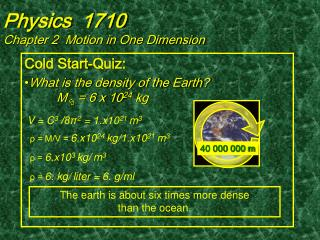DownloadDownload PresentationPhysics 1710 Chapter 2 Motion in One Dimension

# Physics 1710 Chapter 2 Motion in One Dimension

Download Presentation## Physics 1710 Chapter 2 Motion in One Dimension

- - - - - - - - - - - - - - - - - - - - - - - - - - - E N D - - - - - - - - - - - - - - - - - - - - - - - - - - -
##### Presentation Transcript

1. Physics 1710Chapter 2 Motion in One Dimension V = C3 /8π2 = 1.x1021 m3 Cold Start-Quiz: What is the density of the Earth? M = 6 x 1024 kg ρ = M/V =6.x1024 kg/1.x1021 m3 ρ = 6.x103 kg/m3 ρ = 6. kg/liter = 6. g/ml 40 000 000 m The earth is about six times more dense than the ocean.

2. Physics 1710Chapter 2 Motion in One Dimension Foolscap Quiz: What is the volume of the Earth? What is the density of the Earth if its mass is M = 6 x 1024 kg? (Use 1 significant figure.) 40 000 000 m

3. Physics 1710Chapter 2 Motion in One Dimension V = [4π/3]r 3 =2/3 (2π r )3/ (2π )2 = 2/3 C3/ (2π)2 = V = 0.7 •(4. X 10 7 )3/ (2 • 3) 2 = = 0.7 • 6 x 10 22 /(4. X 10 1) = = 1. X 10 21 m 3 = 1. X 10 18 liters ρ= M / V = 6 x 10 24 / 1. X 10 21 kg/ m 3=ρ = 6 x 10 3kg/ m 3 = 6 kg/liter

4. Physics 1710Chapter 2 Motion in One Dimension Galileo Galilei (1564-1642) Pioneered kinematics Discredited Aristotle Discovered moons of Jupiter Argued for Copernican Universe

5. Physics 1710Chapter 2 Motion in One Dimension 1′ Lecture Displacement is the change in position. Velocity is the time rate of displacement. Acceleration is the time rate of change in velocity.

6. Physics 1710Chapter 2 Motion in One Dimension Displacement is the change in position. Δx =xfinal - xinitial Δ is the change “operator”: Change in x =x at end– xat start ΔBalance =Balancefinal - Balanceinitial

7. Physics 1710Chapter 2 Motion in One Dimension Velocity is the time rate of displacement. Average velocity: vx, ave = Δ x / Δt Instantaneous velocity: vx = lim Δt→∞Δx /Δt = dx/dt

8. Physics 1710Chapter 2 Motion in One Dimension Position (m) Time (sec)

9. Δx Δt Physics 1710Chapter 2 Motion in One Dimension Plot it! vx = dx/dt Instantaneous Velocity Position (m) vx, ave = Δx / Δt AverageVelocity Velocity Time (sec)

10. Physics 1710Chapter 2 Motion in One Dimension Acceleration is the time rate of change of velocity. Average acceleration: ax, ave = Δvx / Δt = (vx,final -vx, initial )/ Δt Instantaneous acceleration: ax = lim Δt→∞Δvx / Δt = dvx /dt ax = dvx /dt =d(dxx /dt)/dt = d2x/dt 2

11. Physics 1710Chapter 2 Motion in One Dimension Motion Map From “snap shots” of motion at equal intervals of time determine the displacement, the average velocity and the average acceleration in each case. Uniform motion Accelerated motion

12. vx = dx/dt Physics 1710Chapter 2 Motion in One Dimension Plot them! a2>0 Velocity a1= 0 Position (m) Velocity (m/sec) ax = dvx /dt Time (sec) Time (sec)

13. Physics 1710Chapter 2 Motion in One Dimension Summary: The understanding of acceleration is the key to understanding kinematics. The acceleration of freely falling bodies is constant.